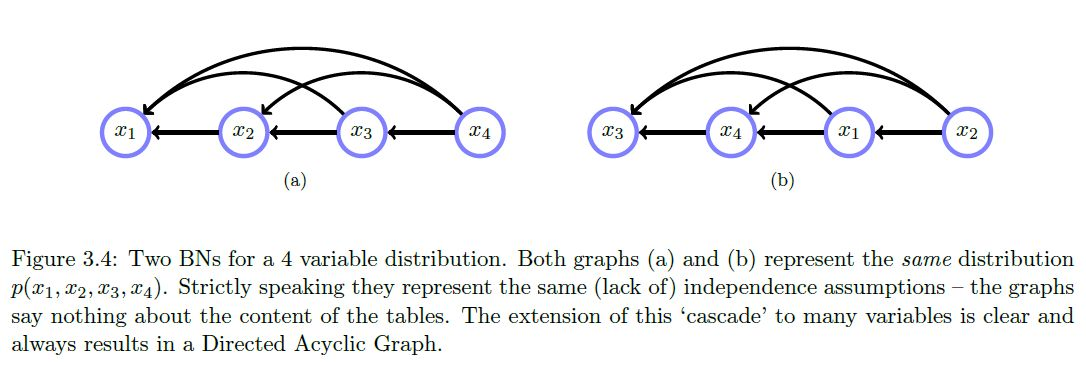Math and science::INF ML AI

# Belief networks

A belief network (called a Bayesian network by Koller) is a grapical model that represents a distribution of the form
$p(x_1, ..., x_D) = \prod_{i=1}^{D} p(x_i \vert pa(x_i)) \\\text{where } pa(x_i) \text{ represents the parental variables of variable } x_i$

Parental variables is just a term for the variables that appear as conditional variables for a given variable.

A belief network represents a distribution as a directed graph where the $$i$$th node corresponds to the factor [...], with directed connections from all [...] nodes to the $$i$$th node.

According to the rules above, a distribution $$p(x_1, x_2, x_3, x_4)$$, one with no independence statements, can be modelled with a cascade graph like so: